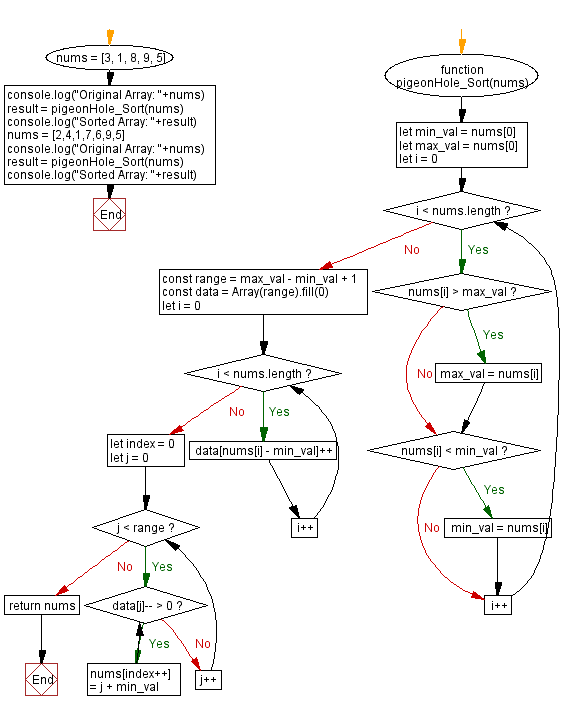# JavaScript Sorting Algorithm: Pigeonhole sort

## JavaScript Sorting Algorithm: Exercise-30 with Solution

Pigeonhole sorting is a sorting algorithm that is suitable for sorting lists of elements where the number n of elements and the length N of the range of possible key values are approximately the same. It requires O(n + N) time. It is similar to counting sort, but differs in that it "moves items twice: once to the bucket array and again to the final destination [whereas] counting sort builds an auxiliary array then uses the array to compute each item's final destination and move the item there."

The pigeonhole algorithm works as follows:

• Given an array of values to be sorted, set up an auxiliary array of initially empty "pigeonholes", one pigeonhole for each key in the range of the keys in the original array.
• Going over the original array, put each value into the pigeonhole corresponding to its key, such that each pigeonhole eventually contains a list of all values with that key.
• Iterate over the pigeonhole array in increasing order of keys, and for each pigeonhole, put its elements into the original array in increasing order.

Write a JavaScript program to sort a list of elements using the Pigeonhole sort algorithm.

Sample Data: Original Array: [3, 1, 8, 9, 5]
Sorted Array: [1, 3, 5, 8, 9]
Original Array: [2, 4, 1, 7, 6, 9, 5]
Sorted Array: [1, 2, 4, 5, 6, 7, 9]

Sample Solution:

JavaScript Code:

``````function pigeonHole_Sort(nums) {
let min_val = nums
let max_val = nums

for (let i = 0; i < nums.length; i++) {
if (nums[i] > max_val) {max_val = nums[i]}
if (nums[i] < min_val) {min_val = nums[i]}
}

const range = max_val - min_val + 1
const data = Array(range).fill(0)

for (let i = 0; i < nums.length; i++) {
data[nums[i] - min_val]++
}

let index = 0

for (let j = 0; j < range; j++) {
while (data[j]-- > 0) {
nums[index++] = j + min_val
}
}
return nums
}
nums = [3, 1, 8, 9, 5]
console.log("Original Array: "+nums)
result = pigeonHole_Sort(nums)
console.log("Sorted Array: "+result)
nums = [2,4,1,7,6,9,5]
console.log("Original Array: "+nums)
result = pigeonHole_Sort(nums)
console.log("Sorted Array: "+result)
```
```

Sample Output:

```"Original Array: 3,1,8,9,5"
"Sorted Array: 1,3,5,8,9"
"Original Array: 2,4,1,7,6,9,5"
"Sorted Array: 1,2,4,5,6,7,9"
```

Flowchart:Live Demo:

See the Pen searching-and-sorting-algorithm-exercise-30 by w3resource (@w3resource) on CodePen.

Improve this sample solution and post your code through Disqus

Previous: Odd - Even sort.
Next: Stooge sort.

What is the difficulty level of this exercise?

Test your Programming skills with w3resource's quiz.

﻿